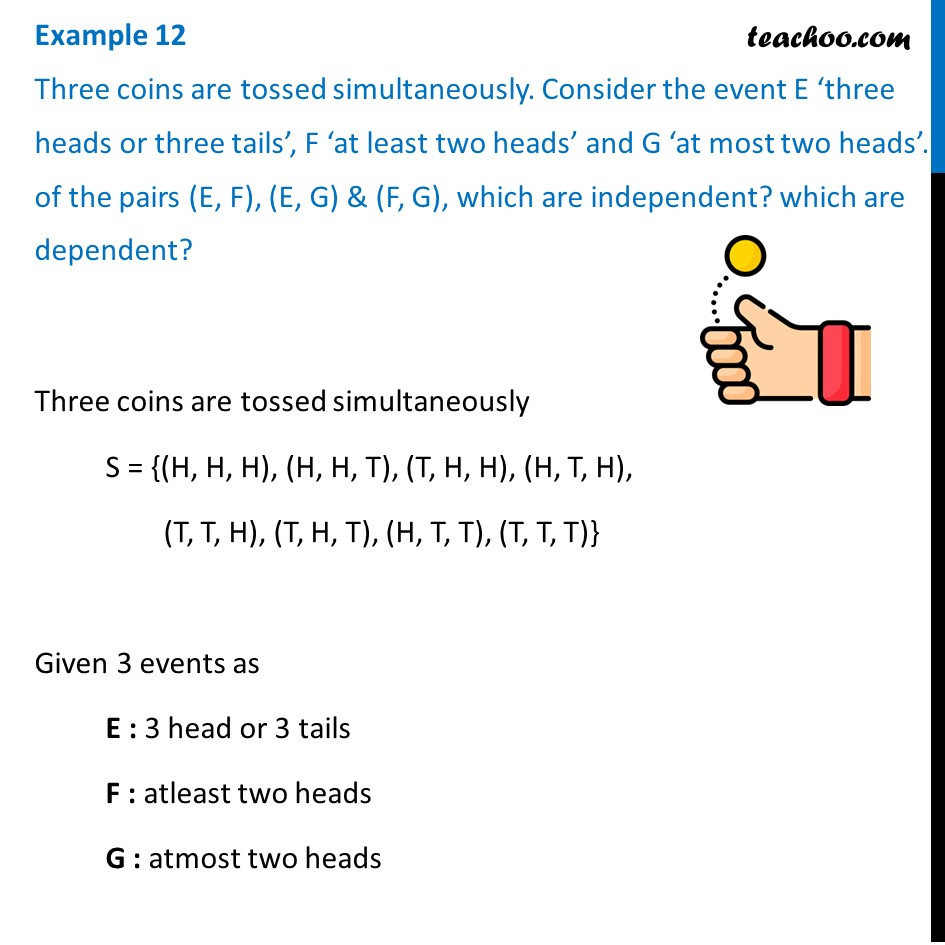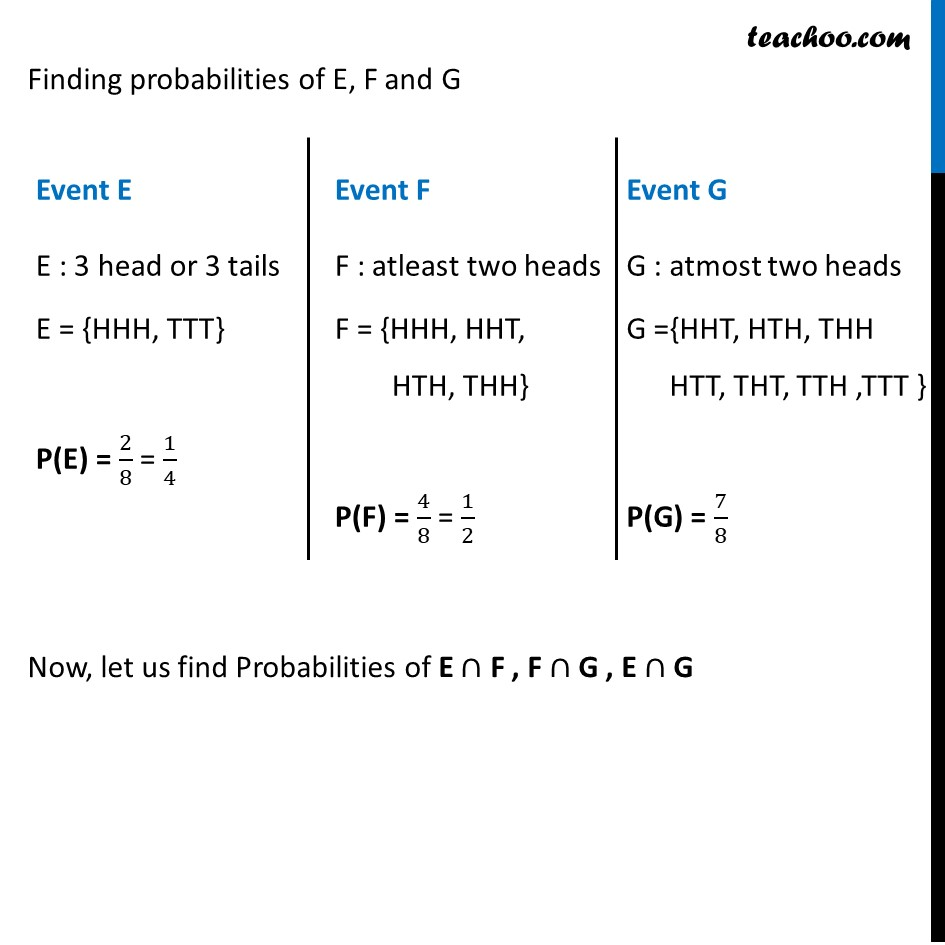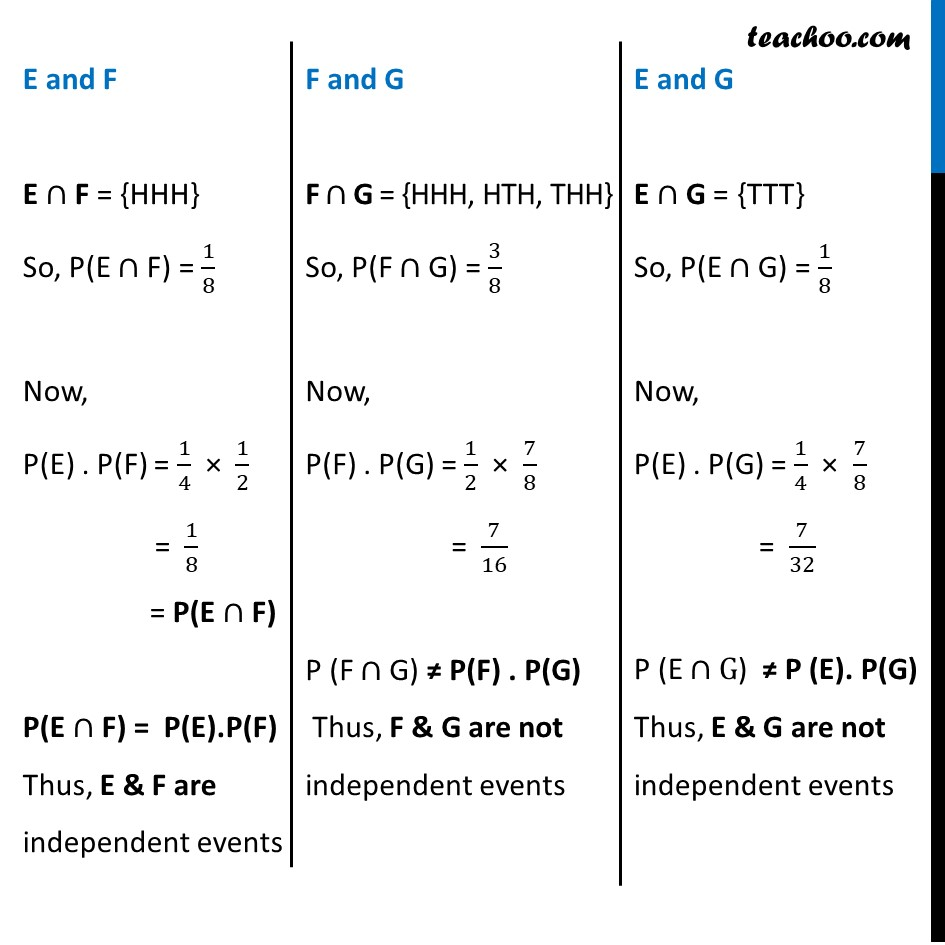Examples

Chapter 13 Class 12 Probability
Serial order wiseLearn in your speed, with individual attention - Teachoo Maths 1-on-1 Class

### Transcript

Example 12 Three coins are tossed simultaneously. Consider the event E ‘three heads or three tails’, F ‘at least two heads’ and G ‘at most two heads’. of the pairs (E, F), (E, G) & (F, G), which are independent? which are dependent? Three coins are tossed simultaneously S = {(H, H, H), (H, H, T), (T, H, H), (H, T, H), (T, T, H), (T, H, T), (H, T, T), (T, T, T)} Given 3 events as E : 3 head or 3 tails F : atleast two heads G : atmost two heads Finding probabilities of E, F and G Event E E : 3 head or 3 tails E = {HHH, TTT} P(E) = 2/8 = 1/4 Event F F : atleast two heads F = {HHH, HHT, HTH, THH} P(F) = 4/8 = 1/2 Event G G : atmost two heads G ={HHT, HTH, THH HTT, THT, TTH ,TTT } P(G) = 7/8 Now, let us find Probabilities of E ∩ F , F ∩ G , E ∩ G E and F E ∩ F = {HHH} So, P(E ∩ F) = 1/8 Now, P(E) . P(F) = 1/4 × 1/2 = 1/8 = P(E ∩ F) P(E ∩ F) = P(E).P(F) Thus, E & F are independent events F and G F ∩ G = {HHH, HTH, THH} So, P(F ∩ G) = 3/8 Now, P(F) . P(G) = 1/2 × 7/8 = 7/16 P (F ∩ G) ≠ P(F) . P(G) Thus, F & G are not independent events E and G E ∩ G = {TTT} So, P(E ∩ G) = 1/8 Now, P(E) . P(G) = 1/4 × 7/8 = 7/32 P (E ∩G) ≠ P (E). P(G) Thus, E & G are not independent events